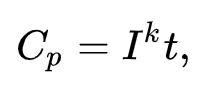The topic about how long does a life of a lead-acid battery will last and what rating should you use depending on what designates to them. It says that if a battery with a rate of 100AH, then it was more or less giving an indication that either 100 hours under a 1 amp load or 1 hour under a 100 amp load will take its life to the fullest. But just what was taught to me back in Calculus, that what I know about logic numbers were about to change. And later one, what I perceive as learnings from the past, suddenly became confusing by now. I know it seems to be quite odd but somehow there’s a point of saying so.

## Battery Capacity - Peukert's Law Explanation

• A battery with an Ah rating always has an hourly rate that the Ah was calculated off.

• The most common hourly rate is 20 hours.

• When you see a battery with a 50AH rating, it is tested at a 20 hour rate (unless it indicated otherwise).

• This simply states that the manufacturer has hit the 50Ah rating on the battery having tested it for 20 hours with an amperage drain of 2.5 amps.

• So logic would tell you that discharging at 8 amps will mean that the battery will last for 6.25 hours (50/8 = 6.25), Right? Wrong!

• Enter Peukert’s Law - The good thing is there is a formula that can provide an exact figure on how long will a lead-acid battery last, even on different kinds of loads. This formula is Peukert’s law. This law states mathematically that when the rate of the discharge increases, the available capacity of the battery decreases. This indicates an inverse relationship between the two factors.

The formula for Peukert’s law:• H (hours), rated discharge time
• C (Ampere-hours), rated capacity at a given discharge rate
• I (Amps), actual discharge current
• k (dimensionless), Peukert constant
• t (hours), actual time to discharge a battery

The formula that we are using is as follows:It stands for the discharge rate at the time to discharge, the new AH rating for that battery based on your discharge.

In this part, you can see that in the situation given H=20 hours, we have C=50AH. We also have I=8 amps as the new discharge current, but k is missing. In this part, it might be difficult for us to arrive at the answer if the constant, k – the Peukert constant is not present. Different batteries have different Peukert constant. The value of k normally lies in the range of 1.1 to 1.3. It can also cover 1.05 to 1.15 for AGM batteries, 1.1 to 1.23 for Gel, and 1.2 to 1.6 for Flooded Batteries. Our aim is to guess at the number or work on finding the Peukert constant of your battery by using the given formula.

The only limitation of Peukert's Law is that the Peukert constant has no way to find the age of the battery given or the temperature at which it is discharge, either way, it will lead to a negative effect on the battery capacity

Going back to our given example, by the use of Peukert's Law, this particular battery has a Peukert constant of 1.3, thus if we supply it to the equation we have the result of an effective capacity of 71.9AH with an amperage drain of 15 amps for 4.79 hours. Then, if we put 15 amp load to the sample battery with a rate of 100AH at 20 hour rate, you will come up with a 71.9AH battery at a 4.79 rate. The advantage of having this will enable you to figure out when your battery will last for 2 hours less than a person who has figured by setting 100AH rating as their guide. The greater the load of the battery, the less capacity you will have.

If you have reached this part of the article, well done. I hope that you have gained a little bit of information about the lead-acid battery and how to find out the battery capacity of it. Following this rule, you will be able to identify how long the battery will last.